# CBSE Class 9 Mathematics Areas Of Parallelograms And Triangles VBQs

CBSE Class 9 Mathematics Areas Of Parallelograms And Triangles VBQs read and download in pdf. Value Based Questions come in exams for Area in Standard 9 and are easy to learn and helpful in scoring good marks. You can refer to more chapter wise VBQs for Class 9 Area and also get latest topic wise very useful study material as per latest NCERT book for Class 12 Area and all other subjects for free on Studiestoday designed as per latest Grade 9 CBSE, NCERT and KVS syllabus and examination pattern

## VBQ for Class 9 Area Chapter 9 Areas Of Parallelograms And Triangles

Class 9 Area students should refer to the following value based questions with answers for Chapter 9 Areas Of Parallelograms And Triangles in standard 9. These VBQ questions with answers for Grade 9 Area will come in exams and help you to score good marks

### Chapter 9 Areas Of Parallelograms And Triangles VBQ Questions Class 9 Area with Answers

AREAS OF PARALLELOGRAMSAND TRIANGLES

Question. A craft mela is organised by Welfare Association to promote the art and culture of tribal people. The pandal is to be decorated by usin string of bulbs all around the field. There are two options either to arrange it in a rectangular field ABEF or parallelogram ABCD with equal area.(a) What shape of the field should be chosen to minimise the expense of bulb and why ?

(b) Which values are depicted here ?

Perimeter of rectangle < Perimeter of parallelogram

Question. A plot is in the form of a parallelogram ABCD. Owner of this plot wants to build OLD AGE HOME, DISPENSARY, PARK and HEALTH CENTRE for elderly people as shown in the fig. P is a point on the diagonal BD.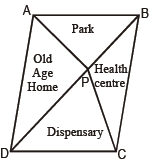(a) Prove that area alloted to old age home and dispensary is same.

(b) Which values are depicted here ?

Diagonals AC & BD of a | |gmABCD bisect at O.

AO = OC and BO = OD

In ΔAPC, PO is median ( Median divides a triangle in two triangles equal in area)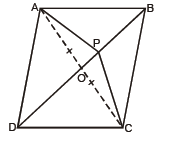therefor,  ar (APO) = ar (CPO)                                        ........... (i)

DO is median

therefor  ar (ADO) = ar(DCO)                                        .......... (ii)

ar (APO) + ar (ADO) = ar (CPO) + ar (DCO)

Question. There was a deserted land near a colony where people used to throw garbage. Colony people united to develop a pond in triangular shape as shown in the fig. The land is in the shape of | |gmABCD. In rest of the portion medicinal plants were grown. If area of parallelogram ABCD is 200 m2.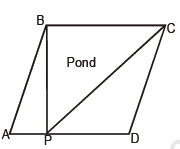(a) Calculate the area where medicinal plants were grown.

(b) Which value is depicted here ?

(b) Environmental protection, cooperation

Question. For ‘Sarva Shiksha Abhiyan’ a rally was organised by a school. Students were given triangular cardboard pieces to write slogans. They divided the triangular shape into three equal parts by drawingmedians as shown in fig.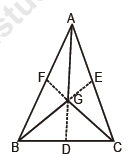(a) Prove that area (AGC) = area (AGB)

= area (BGC)

1/3 area (ABC)

(b) Which values are inculcated through this activity ?

ar ( ΔABD) = ar ( ΔADC) ______ (i)

also ar ( ΔGBD) = ar (GDC) ______ (ii)

subtracting (ii) from (i)

ar ( ΔAGB) = ar ( ΔAGC)

similarly

ar (AGB) = ar (AGC) = ar (GBC)

(b) Cooperation, sincerity

Question. A farmer has a square plot of land where he wants to grow five different crops at a time. On half of the area in the middle he wants to grow wheat but in rest four equal triangular parts he wants to grow different crops.

(a) Explain by diagram how he can divide the area to fulfill his purpose.

(b) By using this crop pattern which values are depicted by the farmer?joining midpoints of sides

(b) Increases fertility of soil, environmental protection, happiness.

Question. There is a plot in a village in the shape of a quadrilateral ABCD. Sarpanch wants to get floor cemented so as to use it for social gatherings and panchayat meetings. Later due to construction of park in the neighbourhood for children they decided to change the shape to triangle ABP. If AC | | DP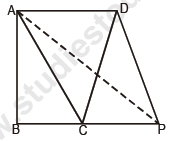Answer: (a) Since AC || DP

ar (ADC) = ar (APC) ..............(i)

( triangles on the same base AC and between same parallels AC & DP are equal in area)

Adding ar (ΔABC) to both sides of (i)

ar (ADC) + ar (ABC) = ar (APC) + ar (ABC)

ar (ABCD) = ar (ABP)

(b) Environmental protection, respecting others views

Question. On National Integration day a poster is to bemade by class IX students of a school. This poster is in the shape of a parallelogram. All religions
should be given equal triangular space to display their teachings.

(a) How will they divide a parallelogram into four triangles equal in area ? Justify it.

(b) What are the values depicted here ?

Answer:  (a) Diagonals of a | | gm divides it into four triangles of equal area.

(b) Unity of nation, co-operation, fraternity

Question. In a class, teacher gave two identical carboard pieces which are in the shape of a parallelogram to two groups. First group was asked to find
area of parallelogramusingAB as base. Then another group was askedto find height (h) of the parallelogram with AD as base.(a) How will they find value of h ?

(b) What are the values involved here ?

Answer: (a) Area of | |gm ABCD

8×3 = 5×h

8×/5 3= h

24/5=h

h = 4.8 cm.

(b) Scientific temper, cooperation

Question. In a class, teacher asks the students to cut a figure from a given parallelogram which has area equal to half the area of parallelogram ABCD. Sunita joins the mid points of opposite sides of parallelogram as shown in the figWhere as Rohan draws a triangle on the same base as shown in fig.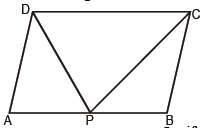(a) State whether two answers are correct. Justify.

(b) What values are depicted from this activity ?

(b) Scientific temper, curiosity, co-operation.

Question. In a rhombus ABCD, AC = 8 cm, then AO = 4 cm.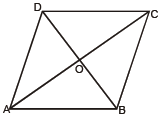The statement shows

(a) Truth value                                        (b) Social value
(c) Environment value                            (d) Cooperation